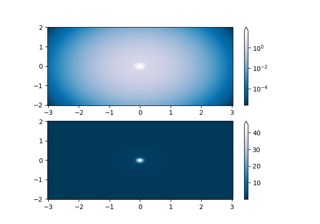# matplotlib.colors.CenteredNorm¶

class matplotlib.colors.CenteredNorm(vcenter=0, halfrange=None, clip=False)[source]

Normalize symmetrical data around a center (0 by default).

Unlike TwoSlopeNorm, CenteredNorm applies an equal rate of change around the center.

Useful when mapping symmetrical data around a conceptual center e.g., data that range from -2 to 4, with 0 as the midpoint, and with equal rates of change around that midpoint.

Parameters: vcenterfloat, default: 0The data value that defines 0.5 in the normalization. halfrangefloat, optionalThe range of data values that defines a range of 0.5 in the normalization, so that vcenter - halfrange is 0.0 and vcenter + halfrange is 1.0 in the normalization. Defaults to the largest absolute difference to vcenter for the values in the dataset.

Examples

This maps data values -2 to 0.25, 0 to 0.5, and 4 to 1.0 (assuming equal rates of change above and below 0.0):

>>> import matplotlib.colors as mcolors
>>> norm = mcolors.CenteredNorm(halfrange=4.0)
>>> data = [-2., 0., 4.]
>>> norm(data)
array([0.25, 0.5 , 1.  ])

__call__(self, value, clip=None)[source]

Normalize value data in the [vmin, vmax] interval into the [0.0, 1.0] interval and return it.

Parameters: valueData to normalize. clipboolIf None, defaults to self.clip (which defaults to False).

Notes

If not already initialized, self.vmin and self.vmax are initialized using self.autoscale_None(value).

__init__(self, vcenter=0, halfrange=None, clip=False)[source]

Normalize symmetrical data around a center (0 by default).

Unlike TwoSlopeNorm, CenteredNorm applies an equal rate of change around the center.

Useful when mapping symmetrical data around a conceptual center e.g., data that range from -2 to 4, with 0 as the midpoint, and with equal rates of change around that midpoint.

Parameters: vcenterfloat, default: 0The data value that defines 0.5 in the normalization. halfrangefloat, optionalThe range of data values that defines a range of 0.5 in the normalization, so that vcenter - halfrange is 0.0 and vcenter + halfrange is 1.0 in the normalization. Defaults to the largest absolute difference to vcenter for the values in the dataset.

Examples

This maps data values -2 to 0.25, 0 to 0.5, and 4 to 1.0 (assuming equal rates of change above and below 0.0):

>>> import matplotlib.colors as mcolors
>>> norm = mcolors.CenteredNorm(halfrange=4.0)
>>> data = [-2., 0., 4.]
>>> norm(data)
array([0.25, 0.5 , 1.  ])

__module__ = 'matplotlib.colors'
__slotnames__ = []
autoscale(self, A)[source]

Set halfrange to max(abs(A-vcenter)), then set vmin and vmax.

autoscale_None(self, A)[source]

Set vmin and vmax.

property halfrange
property vcenter

## Examples using matplotlib.colors.CenteredNorm¶